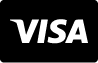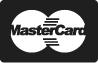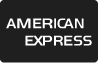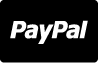Yardim Merkezi

# Stok Fotoğraf - Mathematical formulas. Abstract blue background with Math equations floating on school blackboard. Vector 3D illustration. Symbol of study algebra, arithmetic, physics and exact Sciences

Mathematical formulas. Abstract blue background with Math equations floating on school blackboard. Vector 3D illustration. Symbol of study algebra, arithmetic, physics and exact Sciences
Görsel Kimliği : 109811562
Medya Türü :
Telif hakkı :
7376 x 4166 px | 24.59 " x 13.89 " | 300dpi | JPG
Stok Görsel Anahtar Sözcükleri
Daha Fazla Göster
Daha azını göster
Yardım mı gerekiyor? Özel Hesap Yöneticinizle görüşün
1 866 655 3733